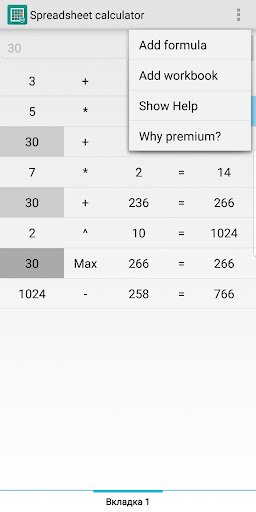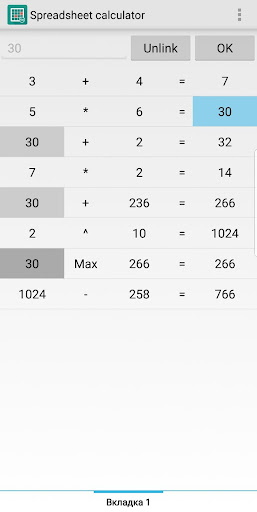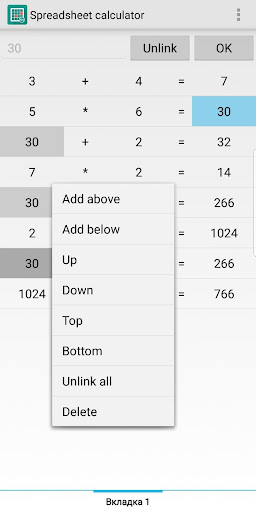In Spreadsheet calculator app each row is a one formula with two operands and result. Each operand you can define or create link to result of previous formula (for example). And when result of previous formula changed - linked operand will be automatically changed. This is so simple! Try it!

- you can create difficult and complex formulas in simple way;
- multiple functions are supported: plus, minus, multiply, divide, percentage, minimum, maximum, root and power
- has workbooks (tabs) for different challenges;
- insert/delete formulas;
- all formulas saved automatically.

Calculator has paid version without ads and without limitations.

In application you can find good interactive help.